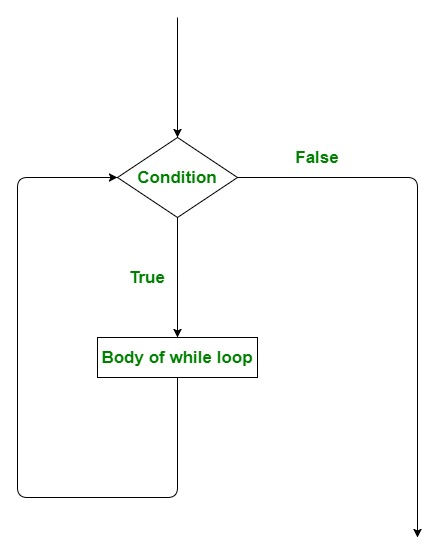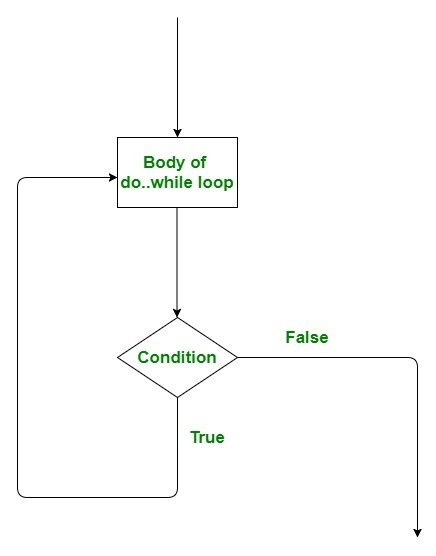GFG App
Open AppBrowser
Continue

# Scala | Loops(while, do..while, for, nested loops)

Looping in programming languages is a feature which facilitates the execution of a set of instructions/functions repeatedly while some condition evaluates to true. Loops make the programmers task simpler. Scala provides the different types of loop to handle the condition based situation in the program. The loops in Scala are :

while Loop

A while loop generally takes a condition in parenthesis. If the condition is True then the code within the body of the while loop is executed. A while loop is used when we don’t know the number of times we want the loop to be executed however we know the termination condition of the loop. It is also known as an entry controlled loop as the condition is checked before executing the loop. The while loop can be thought of as a repeating if statement.
Syntax:

```while (condition)
{
// Code to be executed
}```

Flowchart:• While loop starts with the checking of the condition. If it evaluated to true, then the loop body statements are executed otherwise first statement following the loop is executed. For this reason, it is also called Entry control loop.
• Once the condition is evaluated to true, the statements in the loop body are executed. Normally the statements contain an update value for the variable being processed for the next iteration.
• When the condition becomes false, the loop terminates which marks the end of its life cycle.

Example:

## Scala

 `// Scala program to illustrate while loop` `object` `whileLoopDemo` `{` `    `  `    ``// Main method` `    ``def` `main(args``:` `Array[String])` `    ``{` `        ``var` `x ``=` `1``;`   `        ``// Exit when x becomes greater than 4` `        ``while` `(x ``<=` `4``)` `        ``{` `            ``println(``"Value of x: "` `+ x);`   `            ``// Increment the value of x for` `            ``// next iteration` `            ``x ``=` `x + ``1``;` `        ``}` `    ``}` `}`

Output:

```Value of x: 1
Value of x: 2
Value of x: 3
Value of x: 4```

Infinite While Loop: While loop can execute infinite times which means there is no terminating condition for this loop. In other words, we can say there are some conditions which always remain true, which causes while loop to execute infinite times or we can say it never terminates.
Example: Below program will print the specified statement infinite time and also give the runtime error Killed (SIGKILL) on online IDE.

## Scala

 `// Scala program to illustrate Infinite while loop` `object` `infinitewhileLoopDemo` `{` `    `  `    ``// Main method` `    ``def` `main(args``:` `Array[String])` `    ``{` `        ``var` `x ``=` `1``;`   `        ``// this loop will never terminate` `        ``while` `(x ``<` `5``)` `        ``{` `            ``println(``"GeeksforGeeks"``)` `        ``}` `    ``}` `}`

Output:

```GeeksforGeeks
GeeksforGeeks
GeeksforGeeks
GeeksforGeeks
.
.
.
.```

do..while Loop

A do..while loop is almost same as a while loop. The only difference is that do..while loop runs at least one time. The condition is checked after the first execution. A do..while loop is used when we want the loop to run at least one time. It is also known as the exit controlled loop as the condition is checked after executing the loop.
Syntax:

```do {

// statements to be Executed

} while(condition);```

Flowchart:Example:

## Scala

 `// Scala program to illustrate do..while loop` `object` `dowhileLoopDemo` `{` `    `  `    ``// Main method` `    ``def` `main(args``:` `Array[String])` `    ``{` `        ``var` `a ``=` `10``;`   `        ``// using do..while loop` `        ``do` `        ``{` `            ``print(a + ``" "``);` `            ``a ``=` `a - ``1``;` `        ``}``while``(a ``>` `0``);` `    ``}` `}`

Output:

`10 9 8 7 6 5 4 3 2 1 `

for Loop

for loop has similar functionality as while loop but with different syntax. for loops are preferred when the number of times loop statements are to be executed is known beforehand. There are many variations of “for loop in Scala” which we will discuss in upcoming articles. Basically, it is a repetition control structure which allows the programmer to write a loop that needs to execute a particular number of times.
Example:

## Scala

 `// Scala program to illustrate for loop` `object` `forloopDemo {` `    `  `   ``// Main Method` `   ``def` `main(args``:` `Array[String]) {` `       `  `      ``var` `y ``=` `0``;` `      `  `      ``// for loop execution with range` `      ``for``(y ``<``- ``1` `to ``7``)` `      ``{` `         ``println(``"Value of y is: "` `+ y);` `      ``}` `   ``}` `}`

Output:

```Value of y is: 1
Value of y is: 2
Value of y is: 3
Value of y is: 4
Value of y is: 5
Value of y is: 6
Value of y is: 7```

Nested Loops

The loop which contains a loop inside a loop is known as the nested loop. It can contain the for loop inside a for loop or a while loop inside a while loop. It is also possible that a while loop can contain the for loop and vice-versa.
Example:

## Scala

 `// Scala program to illustrate nested loop` `object` `nestedLoopDemo {` `    `  `  ``// Main Method    ` `  ``def` `main(args``:` `Array[String]) {` `    `  `    ``var` `a ``=` `5``;` `    ``var` `b ``=` `0``;` `    `  `    ``// outer while loop` `    ``while` `(a ``<` `7``) ` `    ``{` `       ``b ``=` `0``;` `       `  `       ``// inner while loop` `       ``while` `(b ``<` `7` `) ` `       ``{` `           `  `           ``// printing the values of a and b` `          ``println(``"Value of a = "` `+a, ``" b = "``+b);` `          ``b ``=` `b + ``1``;` `       ``}` `       `  `       ``// new line` `       ``println()` `       `  `       ``// incrementing the value of a` `       ``a ``=` `a + ``1``;` `       `  `       ``// displaying the updated value of a` `       ``println(``"Value of a Become: "``+a);` `       `  `       ``// new line` `       ``println()` `    ``}`   `  ``}` `}`

Output:

```(Value of a = 5, b = 0)
(Value of a = 5, b = 1)
(Value of a = 5, b = 2)
(Value of a = 5, b = 3)
(Value of a = 5, b = 4)
(Value of a = 5, b = 5)
(Value of a = 5, b = 6)

Value of a Become: 6

(Value of a = 6, b = 0)
(Value of a = 6, b = 1)
(Value of a = 6, b = 2)
(Value of a = 6, b = 3)
(Value of a = 6, b = 4)
(Value of a = 6, b = 5)
(Value of a = 6, b = 6)

Value of a Become: 7```

My Personal Notes arrow_drop_up